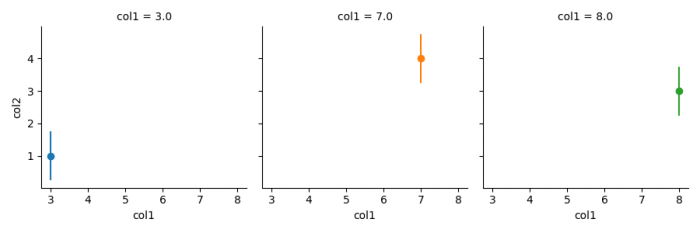# Plotting error bars from a dataframe using Seaborn FacetGrid (Matplotlib)

MatplotlibPythonData Visualization

To plot error bars from a dataframe using Seaborn FacetGrid, we can use following steps −

• Get a two-dimensional, size-mutable, potentially heterogeneous tabular data.
• Multi-plot grid for plotting conditional relationships.
• Apply a plotting function to each facet's subset of the data.
• To display the figure, use show() method.

## Example

import pandas as pd
import seaborn as sns
from matplotlib import pyplot as plt

df = pd.DataFrame({'col1': [3.0, 7.0, 8.0],
'col2': [1.0, 4.0, 3.0]})

g = sns.FacetGrid(df, col="col1", hue="col1")
g.map(plt.errorbar, "col1", "col2", yerr=0.75, fmt='o')

plt.show()

## Output